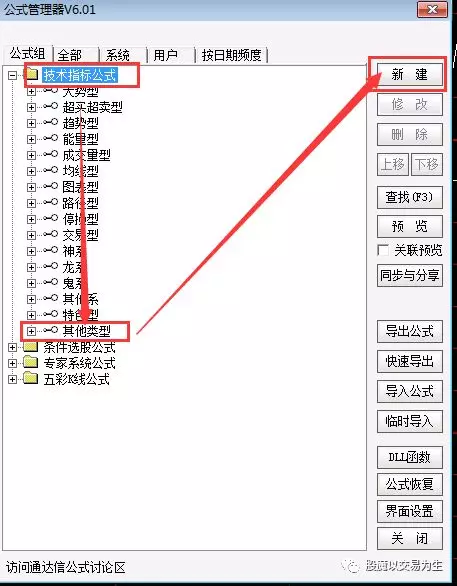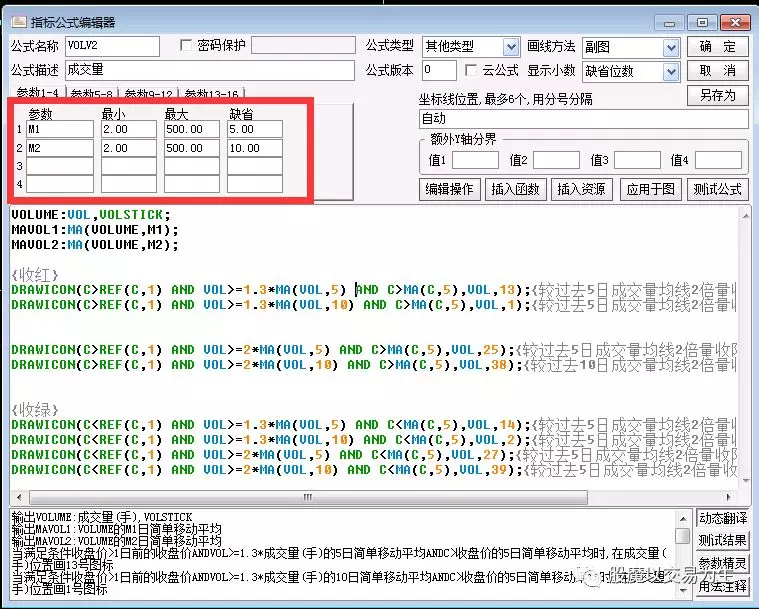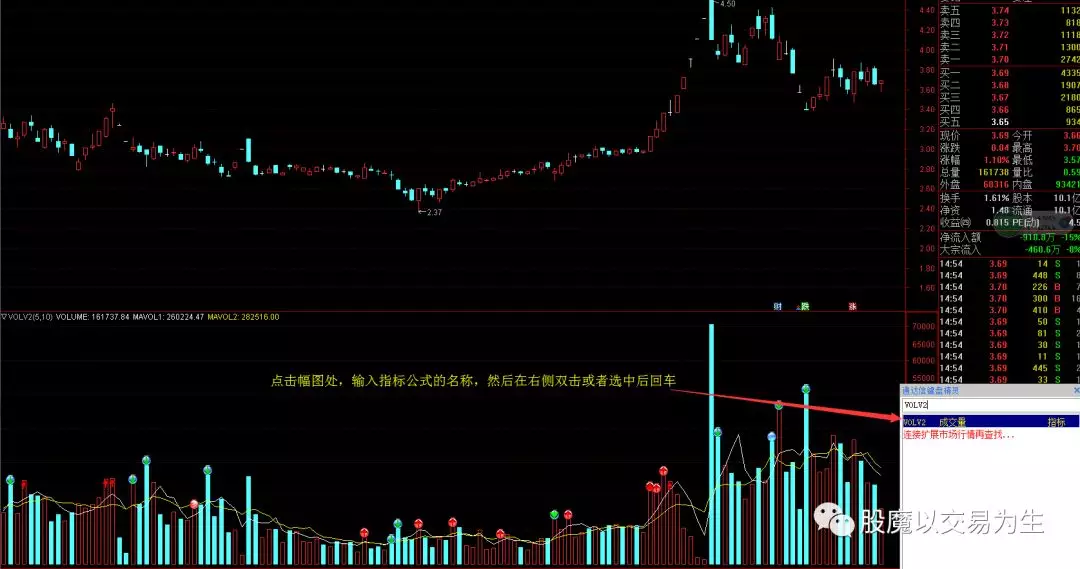成交量公式（付源码）

2019-05-18 22:51:43 技术分析知识库

1.首先，下载通达信软件并安装2.按CTRL+F调出公式管理器3.VOLUME:VOL,VOLSTICK;

MAVOL1:MA(VOLUME,M1);

MAVOL2:MA(VOLUME,M2);

{收红}

DRAWICON(C>REF(C,1) AND VOL>=1.3*MA(VOL,5) AND C>MA(C,5),VOL,13);{较过去5日成交量均线2倍量收阳线，标记红色小球}

DRAWICON(C>REF(C,1) AND VOL>=1.3*MA(VOL,10) AND C>MA(C,5),VOL,1);{较过去10日成交量均线2倍量收阳线，标记红色箭头}

DRAWICON(C>REF(C,1) AND VOL>=2*MA(VOL,5) AND C>MA(C,5),VOL,25);{较过去5日成交量均线2倍量收阳线，标记红色钻石}

DRAWICON(C>REF(C,1) AND VOL>=2*MA(VOL,10) AND C>MA(C,5),VOL,38);{较过去10日成交量均线2倍量收阳线，标记红三角}

{收绿}

DRAWICON(C<REF(C,1) AND VOL>=1.3*MA(VOL,5) AND C<MA(C,5),VOL,14);{较过去5日成交量均线2倍量收阴线，标记蓝色小球}

DRAWICON(C<REF(C,1) AND VOL>=1.3*MA(VOL,10) AND C<MA(C,5),VOL,2);{较过去10日成交量均线2倍量收阴线，标记绿色箭头}

DRAWICON(C<REF(C,1) AND VOL>=2*MA(VOL,5) AND C<MA(C,5),VOL,27);{较过去5日成交量均线2倍量收阴线，标记绿色钻石}

DRAWICON(C<REF(C,1) AND VOL>=2*MA(VOL,10) AND C<MA(C,5),VOL,39);{较过去10日成交量均线2倍量收阴线，标记绿三角};

4.如何调用？[!--temp.sitename--]声明：资讯来源于互联网，属作者个人观点，仅供投资者参考，并不构成投资建议。投资者据此操作，风险自担。